hcurl## hcurl

بازدید : 480 جمعه 12 دي 1393

`(hcurl(uexpr,vexpr`

Calculates the vertical component of the curl (ie, vorticity) at each grid point using finite differencing on the grids provided. It is assumed that `uexpr` gives the U Wind component, and that `vexpr` provides the V Wind component.

### Usage Notes

1. The alghorithm used for the finite difference calculation is described as an Example for the `cdiff` function.

2. The function assumes an X-Y varying dimension environment, and will not operate unless that is the case. The `define` command can be used in conjunction with the `hcurl` function to create 3 or 4 dimensional fields of vorticity, from which vertical cross-sections could be displayed.

3. The boundaries of the grid are set to missing.

4. The radius of the earth used in the calculation is in meters; thus the units of the wind expressions provided would normally be m/s.

### Examples

1. To display the vorticity:

d hcurl(u,v)

2. If you want to display a vertical cross section of vorticity, you first need to calculate it over a 3-Dimensional region:

`set lon 0 360 `
`set lat -90 90 `
`set lev 1000 100 `
`define vort = hcurl(u,v) `
`set lon -90 `
`display vort `
مطالب مرتبط
ارسال نظر برای این مطلب

نام
ایمیل (منتشر نمی‌شود) (لازم)
وبسایت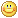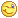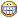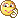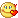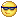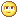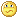نظر خصوصی
مشخصات شما ذخیره شود ؟ [حذف مشخصات] [شکلک ها]کد امنیتی
اطلاعات کاربری
 نام کاربری : رمز عبور :
• فراموشی رمز عبور؟
• لینک دوستان
آرشیو
آمار سایت
• کل مطالب : 155
• کل نظرات : 0
• افراد آنلاین : 1
• تعداد اعضا : 4
• آی پی امروز : 20
• آی پی دیروز : 19
• بازدید امروز : 70
• باردید دیروز : 268
• گوگل امروز : 1
• گوگل دیروز : 0
• بازدید هفته : 388
• بازدید ماه : 1,461
• بازدید سال : 35,866
• بازدید کلی : 156,918
• کدهای اختصاصی